## Meissel's Formula

A modification of Legendre's Formula for the Prime Counting Function. It starts with(1)

whereis the Floor Function,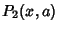is the number of Integerswith, andis the number of Integerswith. Identities satisfied by the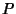s include(2)

forand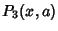(3)

Meissel's formula is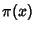(4)

where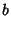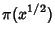(5)(6)

Taking the derivation one step further yields Lehmer's Formula.

Riesel, H. Meissel's Formula.'' Prime Numbers and Computer Methods for Factorization, 2nd ed. Boston, MA: Birkhäuser, p. 12, 1994.